# 十五、使用 RNN 和 CNN 处理序列

• 不稳定梯度（换句话说，在第 11 章中讨论的梯度消失/爆炸），可以使用多种方法缓解，包括循环丢弃和循环层归一化。

• 有限的短期记忆，可以通过 LSTM 和 GRU 单元延长。

RNN 不是唯一能处理序列数据的神经网络：对于小序列，常规紧密网络也可以；对于长序列，比如音频或文本，卷积神经网络也可以。我们会讨论这两种方法，本章最后会实现一个 WaveNet：这是一种 CNN 架构，可以处理上万个时间步的序列。在第 16 章，还会继续学习 RNN，如何使用 RNN 来做自然语言处理，和基于注意力机制的新架构。

## 循环神经元和层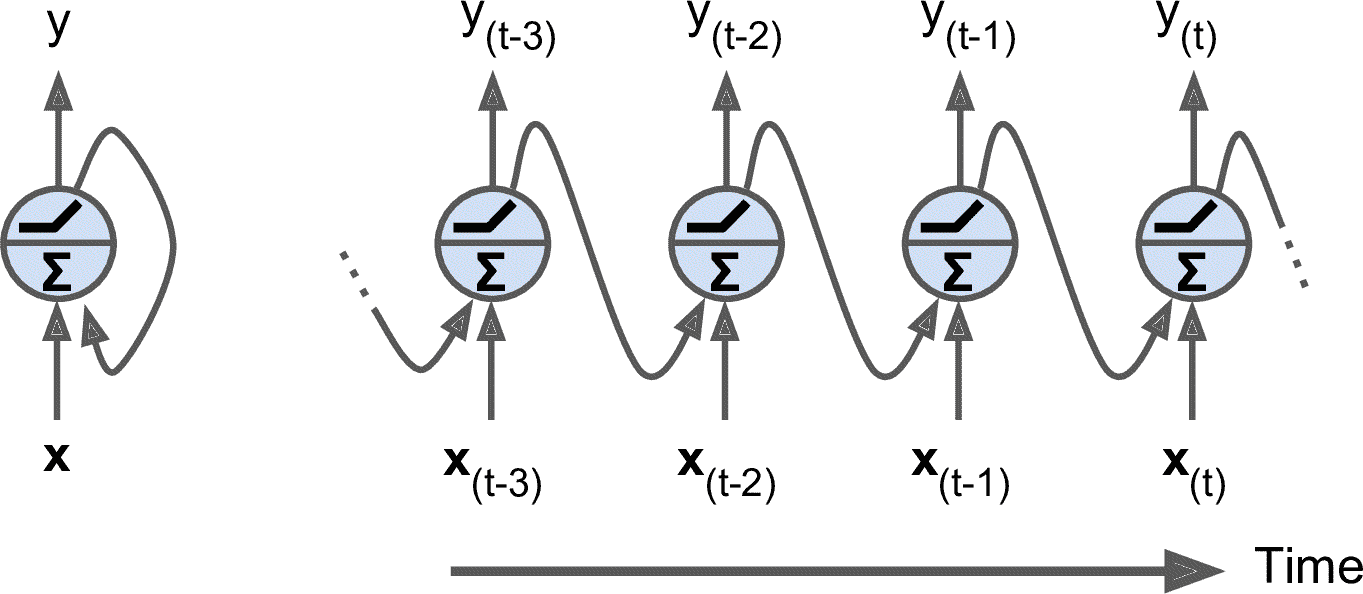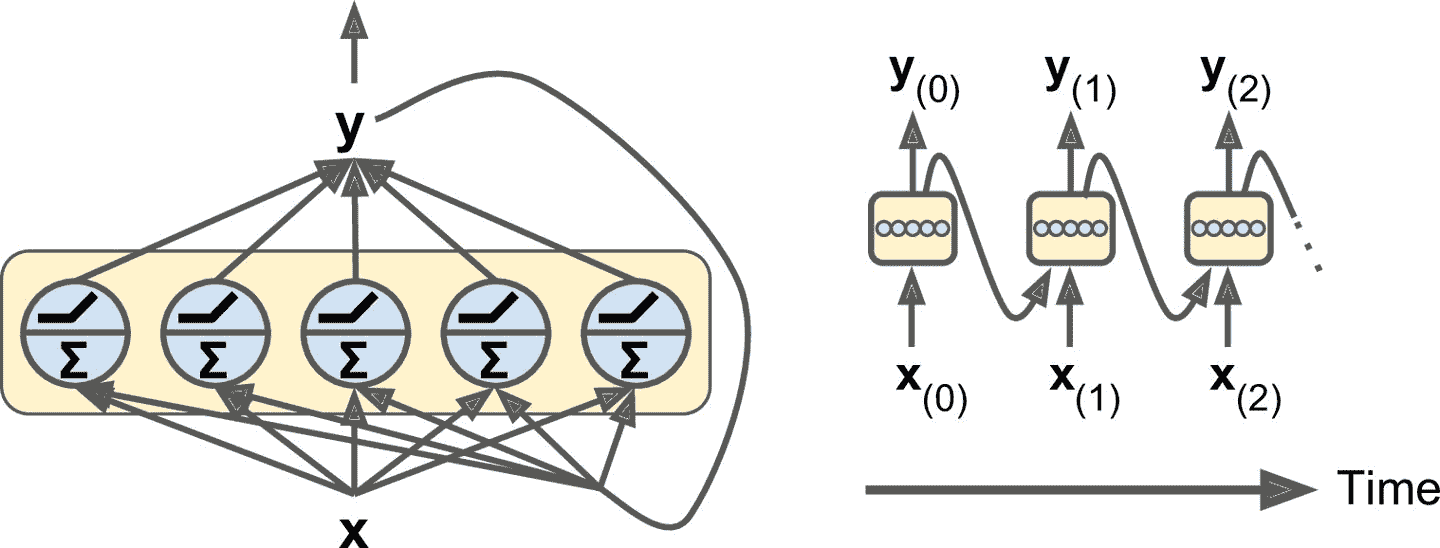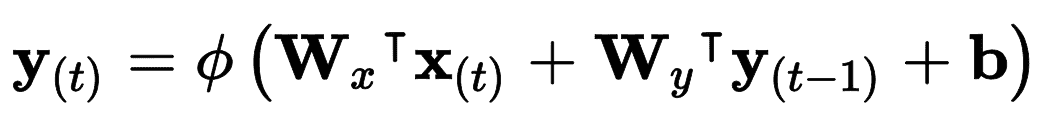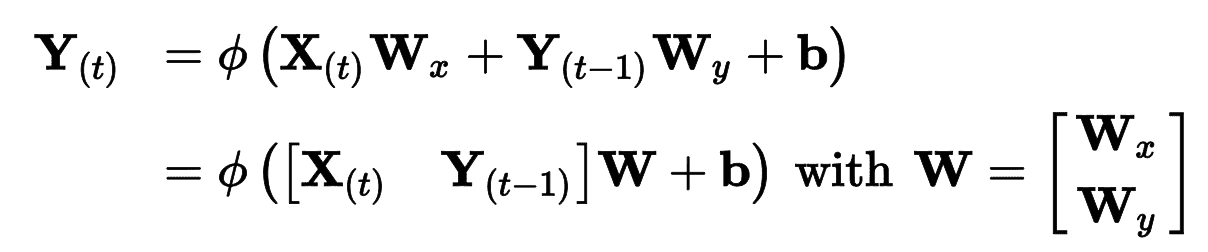• Y[t]m × n_neurons矩阵，包含在小批次中每个实例在时间步t的层输出（m是小批次中的实例数，n_neurons是神经元数）。
• X[t]m × n_inputs矩阵，包含所有实例的输入 （n_inputs是输入特征的数量）。
• W[x]n_inputs × n_neurons矩阵，包含当前时间步的输入的连接权重。
• W[y]n_neurons × n_neurons矩阵，包含上一个时间步的输出的连接权重。
• b是大小为n_neurons的向量，包含每个神经元的偏置项。
• 权重矩阵W[x]W[y]通常纵向连接成一个权重矩阵W，形状为(n_inputs + n_neurons) × n_neurons（见公式 15-2 的第二行）

### 记忆单元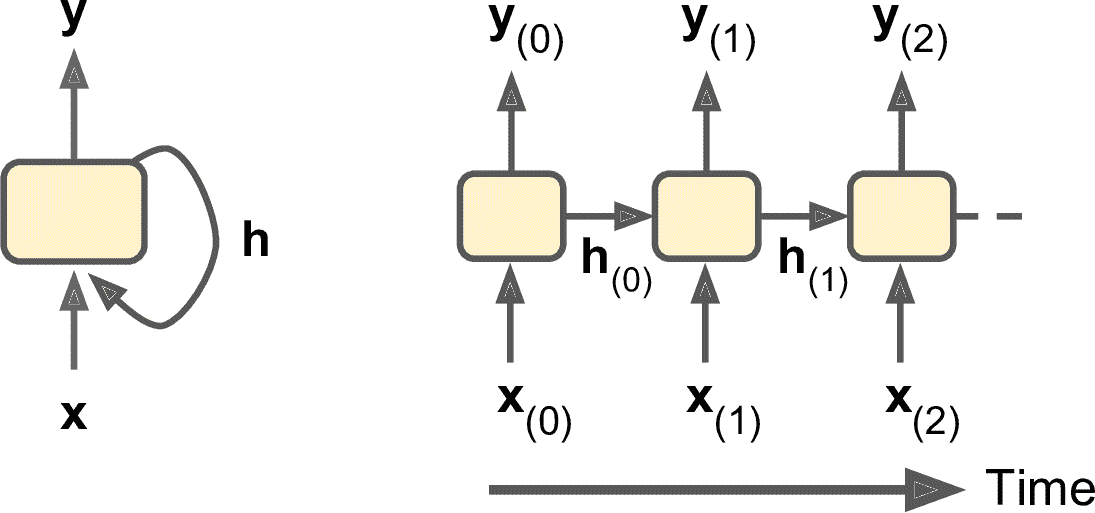## 输入和输出序列

RNN 可以同时输入序列并输出序列（见图 15-4，左上角的网络）。这种序列到序列的网络可以有效预测时间序列（如股票价格）：输入过去N天价格，则输出向未来移动一天的价格（即，从N - 1天前到明天）。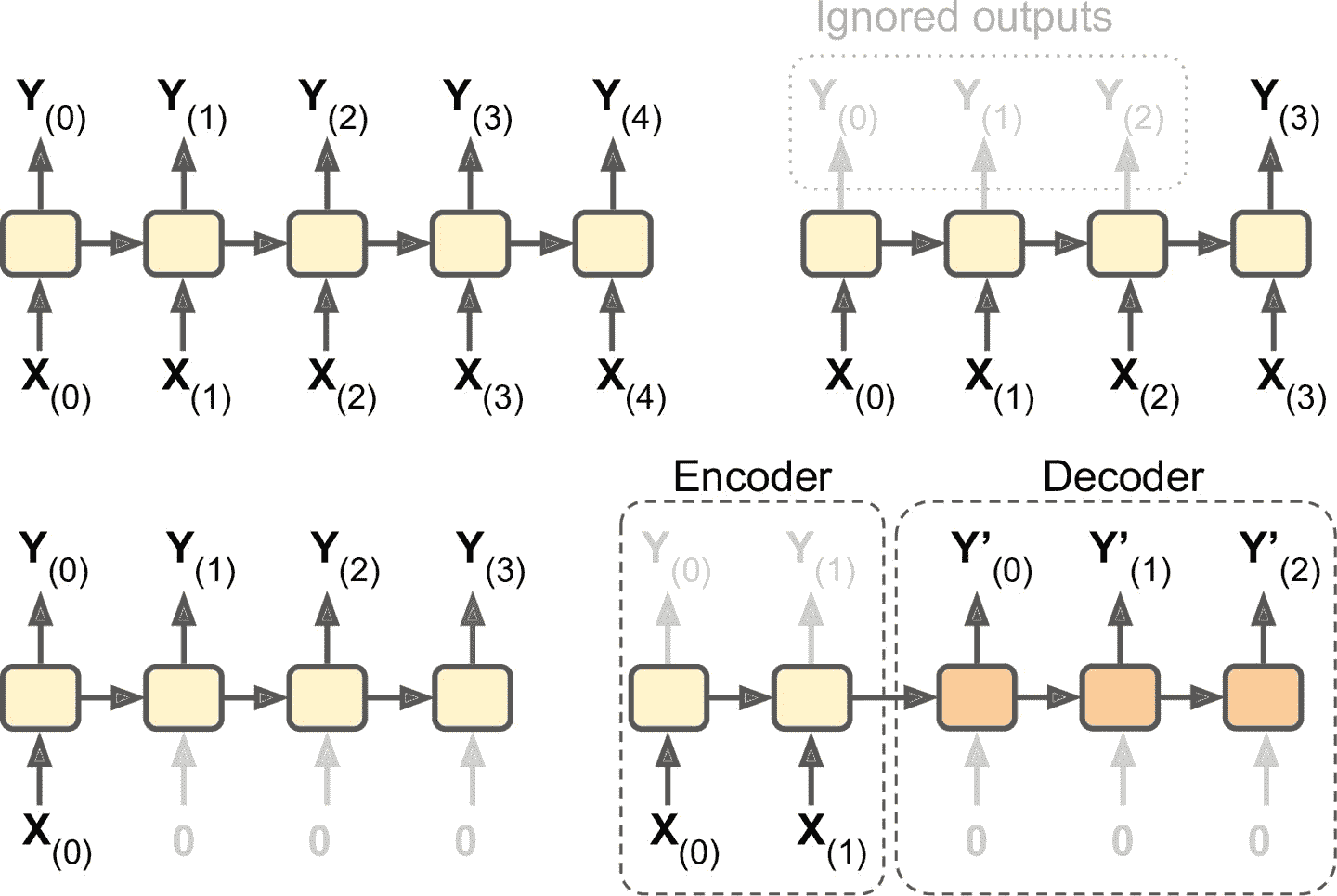## 训练 RNN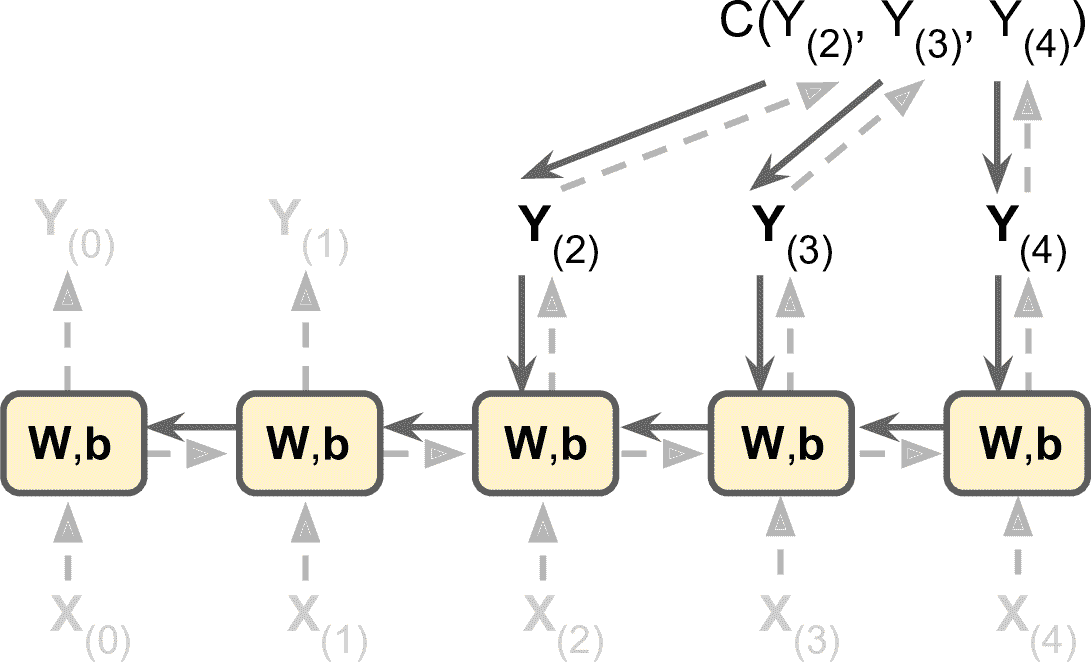## 预测时间序列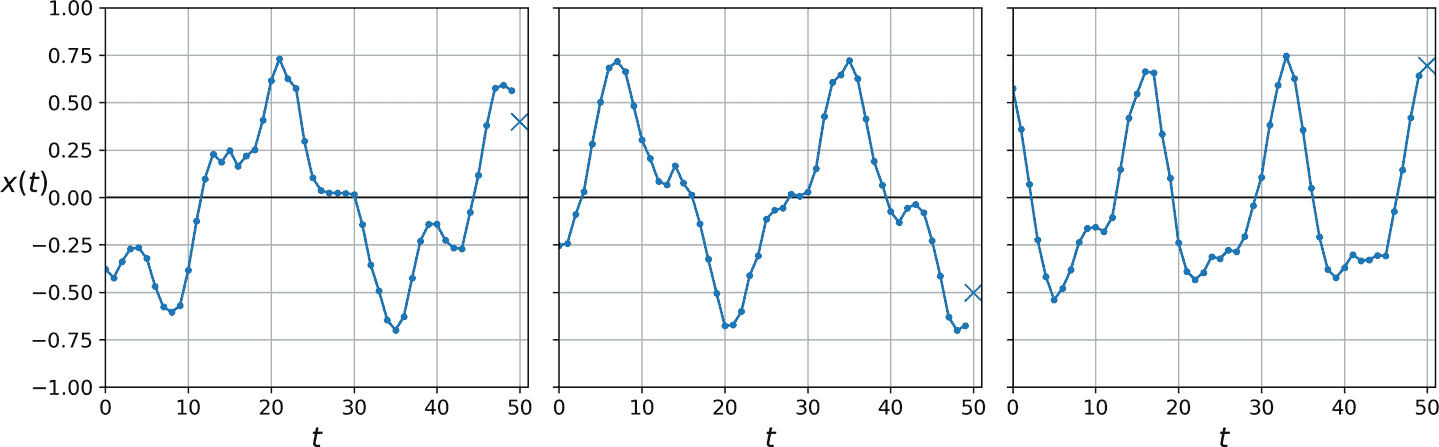def generate_time_series(batch_size, n_steps):
freq1, freq2, offsets1, offsets2 = np.random.rand(4, batch_size, 1)
time = np.linspace(0, 1, n_steps)
series = 0.5 * np.sin((time - offsets1) * (freq1 * 10 + 10))  #   wave 1
series += 0.2 * np.sin((time - offsets2) * (freq2 * 20 + 20)) # + wave 2
series += 0.1 * (np.random.rand(batch_size, n_steps) - 0.5)   # + noise
return series[..., np.newaxis].astype(np.float32)


n_steps = 50
series = generate_time_series(10000, n_steps + 1)
X_train, y_train = series[:7000, :n_steps], series[:7000, -1]
X_valid, y_valid = series[7000:9000, :n_steps], series[7000:9000, -1]
X_test, y_test = series[9000:, :n_steps], series[9000:, -1]


X_train包含 7000 个时间序列（即，形状是 [7000, 50, 1]），X_valid有 2000 个，X_test有 1000 个。因为预测的是单一值，目标值是列向量（y_train的形状是[7000, 1]）。

### 基线模型

>>> y_pred = X_valid[:, -1]
>>> np.mean(keras.losses.mean_squared_error(y_valid, y_pred))
0.020211367


model = keras.models.Sequential([
keras.layers.Flatten(input_shape=[50, 1]),
keras.layers.Dense(1)
])


### 实现一个简单 RNN

model = keras.models.Sequential([
keras.layers.SimpleRNN(1, input_shape=[None, 1])
])


### 深度 RNN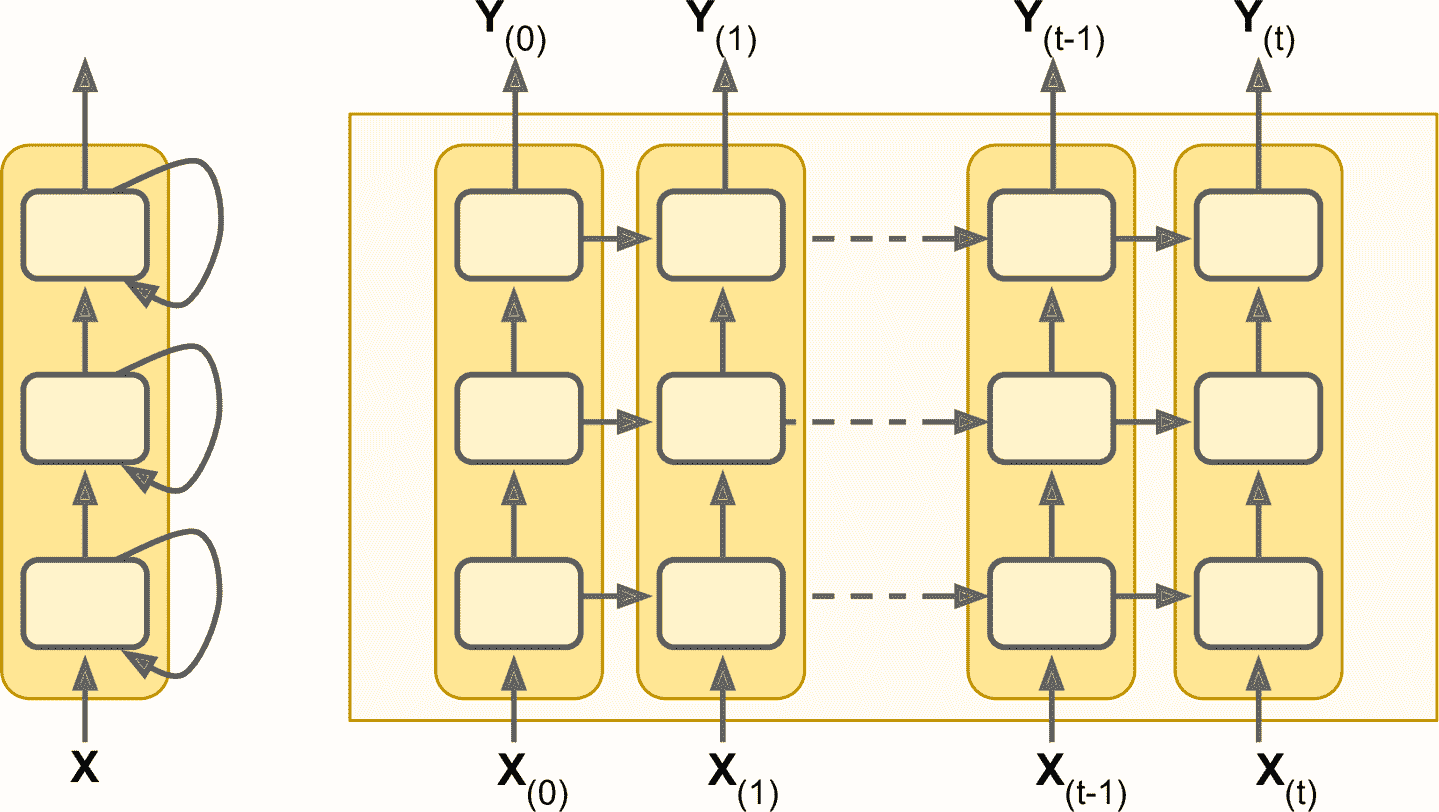tf.keras实现深度 RNN 相当容易：将循环层堆起来就成。在这个例子中，我们使用三个SimpleRNN层（也可以添加其它类型的循环层，比如 LSTM 或 GRU）：

model = keras.models.Sequential([
keras.layers.SimpleRNN(20, return_sequences=True, input_shape=[None, 1]),
keras.layers.SimpleRNN(20, return_sequences=True),
keras.layers.SimpleRNN(1)
])


model = keras.models.Sequential([
keras.layers.SimpleRNN(20, return_sequences=True, input_shape=[None, 1]),
keras.layers.SimpleRNN(20),
keras.layers.Dense(1)
])


### 提前预测几个时间步

series = generate_time_series(1, n_steps + 10)
X_new, Y_new = series[:, :n_steps], series[:, n_steps:]
X = X_new
y_pred_one = model.predict(X[:, step_ahead:])[:, np.newaxis, :]
X = np.concatenate([X, y_pred_one], axis=1)

Y_pred = X[:, n_steps:]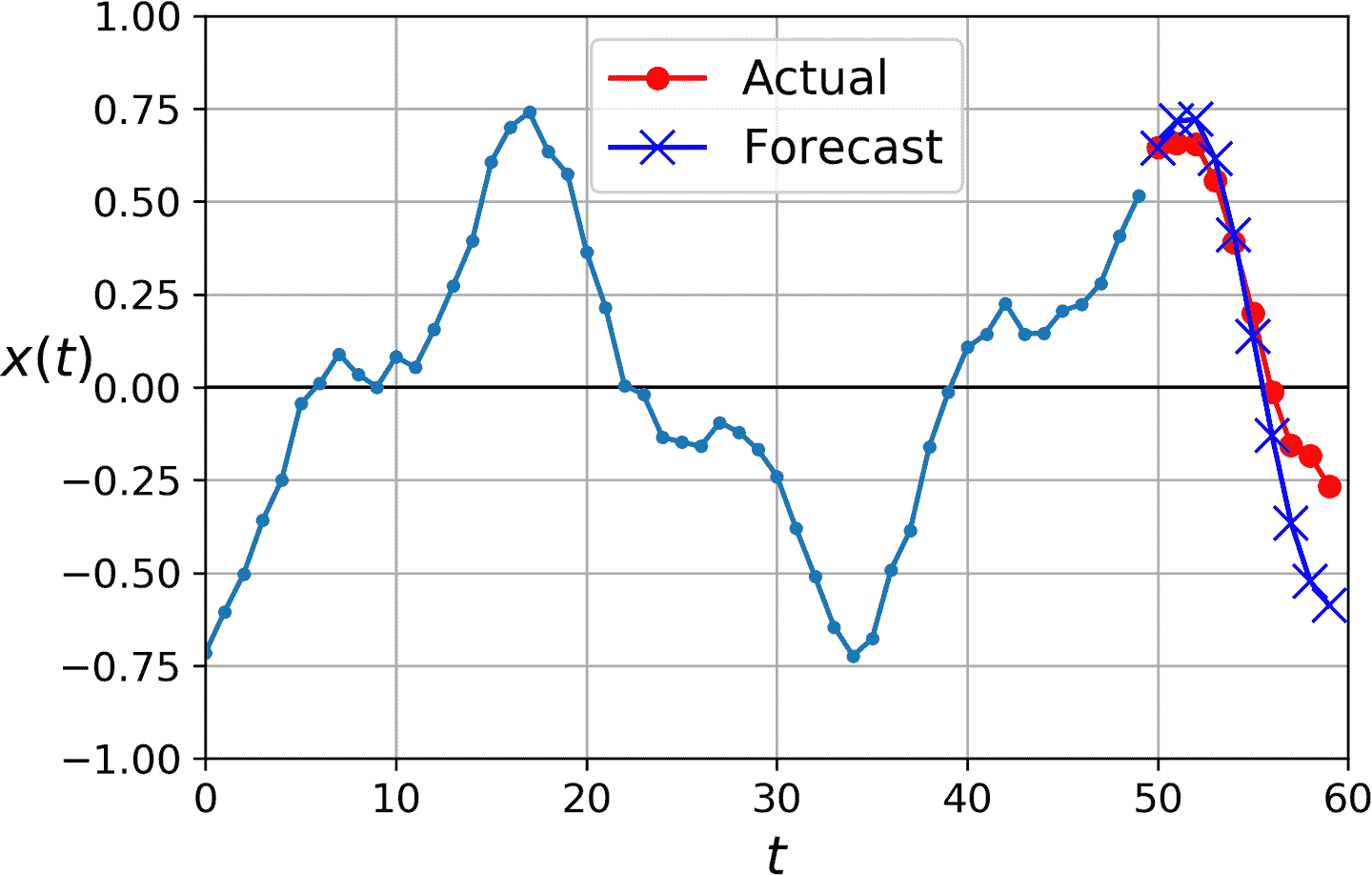series = generate_time_series(10000, n_steps + 10)
X_train, Y_train = series[:7000, :n_steps], series[:7000, -10:, 0]
X_valid, Y_valid = series[7000:9000, :n_steps], series[7000:9000, -10:, 0]
X_test, Y_test = series[9000:, :n_steps], series[9000:, -10:, 0]


model = keras.models.Sequential([
keras.layers.SimpleRNN(20, return_sequences=True, input_shape=[None, 1]),
keras.layers.SimpleRNN(20),
keras.layers.Dense(10)
])


Y_pred = model.predict(X_new)


Y = np.empty((10000, n_steps, 10)) # each target is a sequence of 10D vectors
for step_ahead in range(1, 10 + 1):
Y_train = Y[:7000]
Y_valid = Y[7000:9000]
Y_test = Y[9000:]


model = keras.models.Sequential([
keras.layers.SimpleRNN(20, return_sequences=True, input_shape=[None, 1]),
keras.layers.SimpleRNN(20, return_sequences=True),
keras.layers.TimeDistributed(keras.layers.Dense(10))
])


def last_time_step_mse(Y_true, Y_pred):
return keras.metrics.mean_squared_error(Y_true[:, -1], Y_pred[:, -1])

model.compile(loss="mse", optimizer=optimizer, metrics=[last_time_step_mse])


## 处理长序列

### 应对不稳定梯度

class LNSimpleRNNCell(keras.layers.Layer):
def __init__(self, units, activation="tanh", **kwargs):
super().__init__(**kwargs)
self.state_size = units
self.output_size = units
self.simple_rnn_cell = keras.layers.SimpleRNNCell(units,
activation=None)
self.layer_norm = keras.layers.LayerNormalization()
self.activation = keras.activations.get(activation)
def call(self, inputs, states):
outputs, new_states = self.simple_rnn_cell(inputs, states)
norm_outputs = self.activation(self.layer_norm(outputs))
return norm_outputs, [norm_outputs]


model = keras.models.Sequential([
keras.layers.RNN(LNSimpleRNNCell(20), return_sequences=True,
input_shape=[None, 1]),
keras.layers.RNN(LNSimpleRNNCell(20), return_sequences=True),
keras.layers.TimeDistributed(keras.layers.Dense(10))
])


## LSTM 单元

model = keras.models.Sequential([
keras.layers.LSTM(20, return_sequences=True, input_shape=[None, 1]),
keras.layers.LSTM(20, return_sequences=True),
keras.layers.TimeDistributed(keras.layers.Dense(10))
])


model = keras.models.Sequential([
keras.layers.RNN(keras.layers.LSTMCell(20), return_sequences=True,
input_shape=[None, 1]),
keras.layers.RNN(keras.layers.LSTMCell(20), return_sequences=True),
keras.layers.TimeDistributed(keras.layers.Dense(10))
])


LSTM 单元的工作机制是什么呢？图 15-9 展示了 LSTM 单元的结构。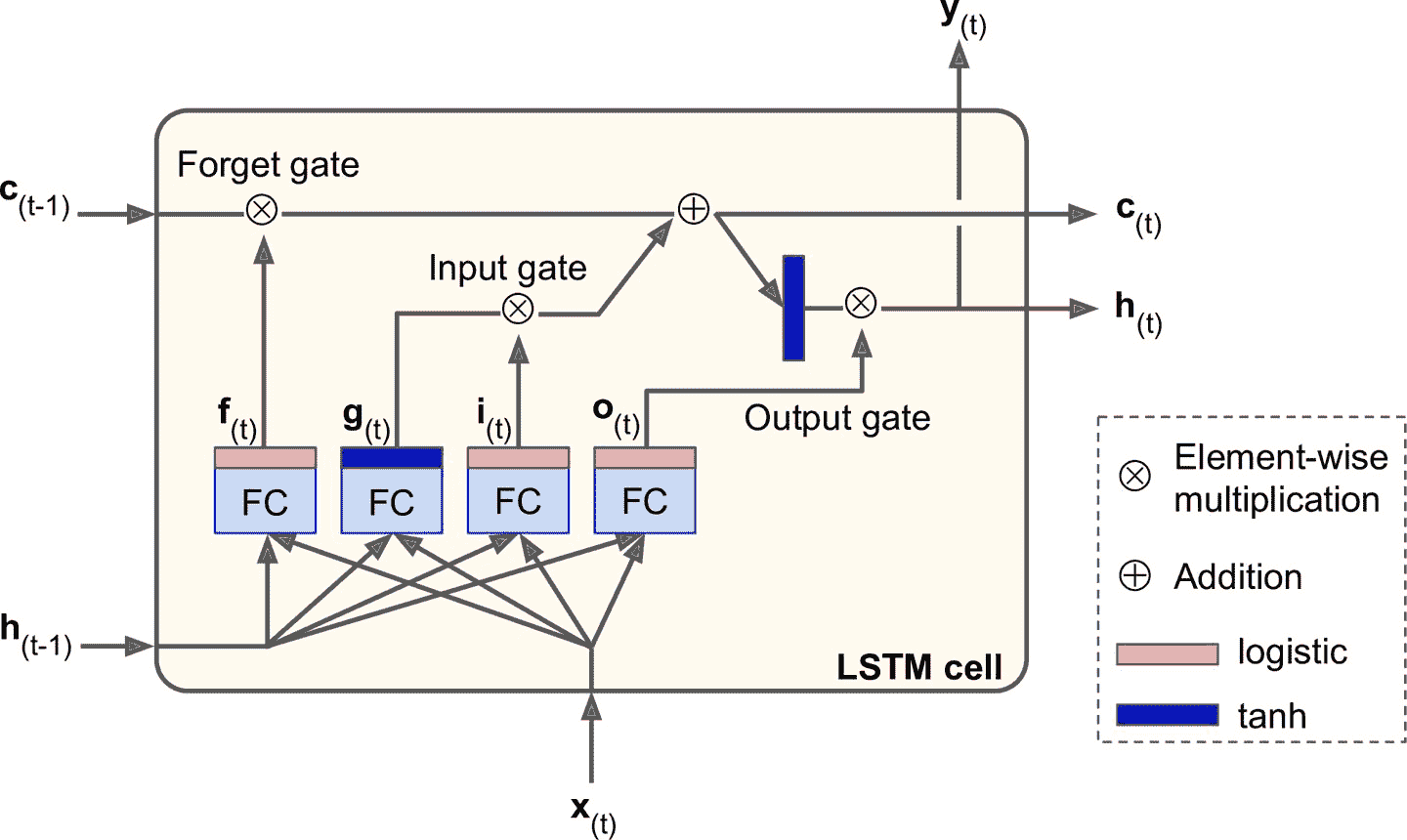• 输出g[t]的层是主要层。它的常规任务是分析当前的输入x[t]和前一时刻的短时状态h[t-1]。基本单元中与这种结构一样，直接输出了h[t]y[t]。相反的，LSTM 单元中的该层的输出不会直接出去，儿是将最重要的部分保存在长期状态中（其余部分丢掉）。

• 其它三个全连接层被是门控制器（gate controller）。其采用 Logistic 作为激活函数，输出范围在 0 到 1 之间。可以看到，这三个层的输出提供给了逐元素乘法操作，当输入为 0 时门关闭，输出为 1 时门打开。具体讲：

• 遗忘门（由f[t]控制）决定哪些长期记忆需要被删除；

• 输入门（由i[t]控制） 决定哪部分g[t]应该被添加到长时状态中。

• 输出门（由o[t]控制）决定长时状态的哪些部分要读取和输出为h[t]y[t]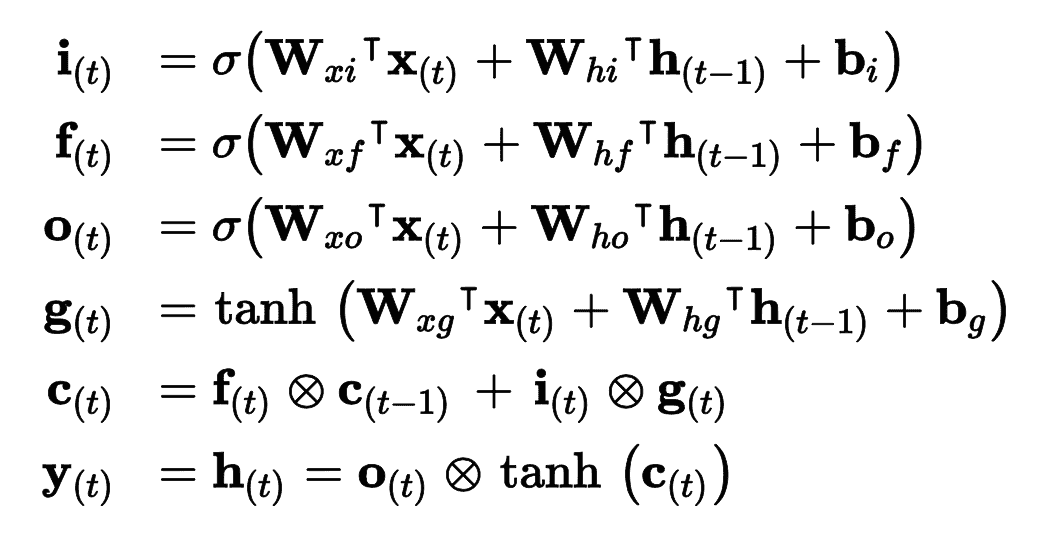• W[xi]W[xf]W[xo]W[xg]是四个全连接层连接输入向量X[t]的权重。

• W[hi]W[hf]W[ho]W[hg]是四个全连接层连接上一时刻的短时状态h[t - 1]的权重。

• b[i]b[f]b[o]b[g]是全连接层的四个偏置项。需要注意的是 TensorFlow 将b[f]初始化为全 1 向量，而非全 0。这样可以保证在训练状态开始时，忘掉所有东西。

### 窥孔连接

Keras 中，LSTM层基于keras.layers.LSTMCell单元，后者目前还不支持窥孔。但是，试验性的tf.keras.experimental.PeepholeLSTMCell支持，所以可以创建一个keras.layers.RNN层，向构造器传入PeepholeLSTMCell

LSTM 有多种其它变体，其中特别流行的是 GRU 单元。

### GRU 单元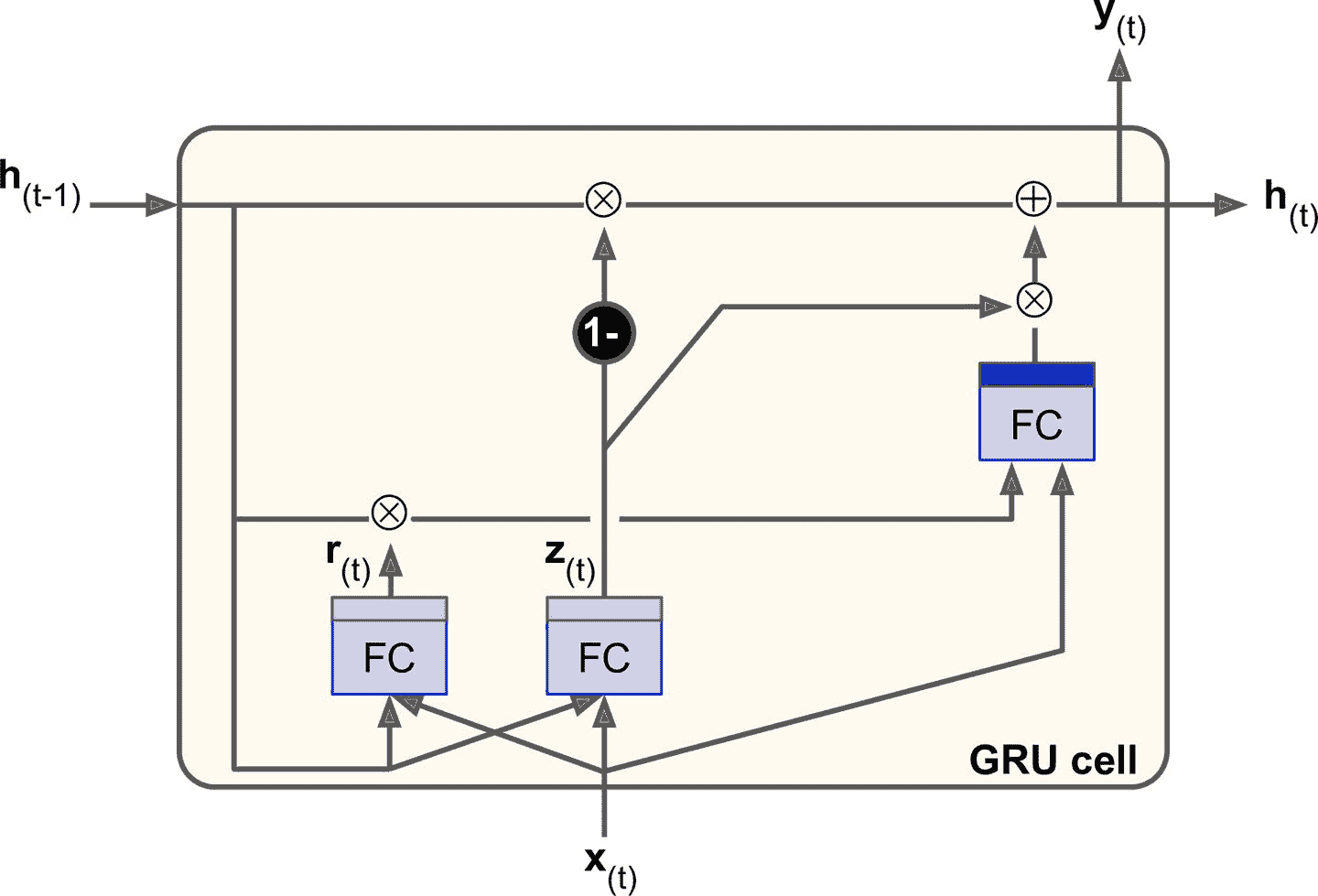GRU 单元是 LSTM 单元的简化版本，能实现同样的性能（这也说明了为什么它能越来越流行）。简化主要在一下几个方面：

• 长时状态和短时状态合并为一个向量h[t]

• 用一个门控制器z[t]控制遗忘门和输入门。如果门控制器输出 1，则遗忘门打开（= 1），输入门关闭（1 - 1 = 0）。如果输出 0，则相反。换句话说，如果当有记忆要存储，那么就必须先在其存储位置删掉该处记忆。这构成了 LSTM 本身的常见变体。

• GRU 单元取消了输出门，每个时间步输出全态向量。但是，增加了一个控制门r[t]来控制前一状态的哪些部分呈现给主层g[t]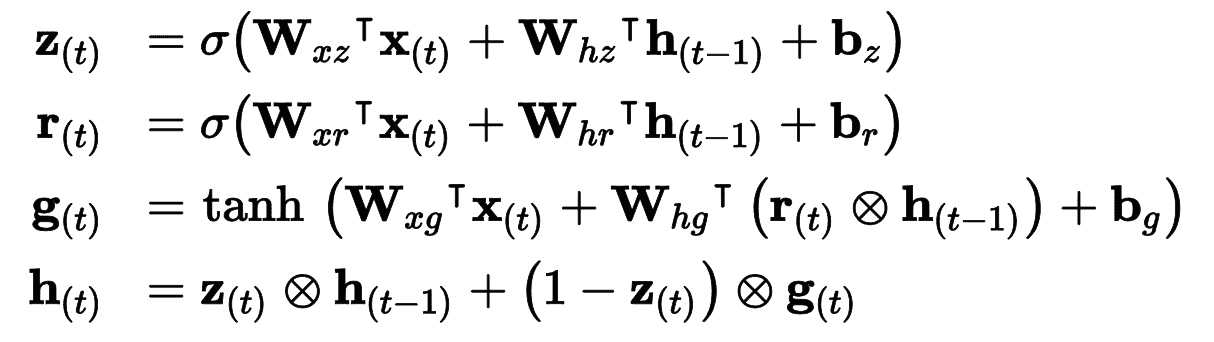Keras 提供了keras.layers.GRU层（基于keras.layers.GRUCell记忆单元）；使用时，只需将SimpleRNNLSTM替换为GRU

LSTM 和 GRU 是 RNN 取得成功的主要原因之一。尽管它们相比于简单 RNN 可以处理更长的序列了，还是有一定程度的短时记忆，序列超过 100 时，比如音频、长时间序列或长序列，学习长时模式就很困难。应对的方法之一，是使用缩短输入序列，例如使用 1D 卷积层。

### 使用 1D 卷积层处理序列

model = keras.models.Sequential([
input_shape=[None, 1]),
keras.layers.GRU(20, return_sequences=True),
keras.layers.GRU(20, return_sequences=True),
keras.layers.TimeDistributed(keras.layers.Dense(10))
])

history = model.fit(X_train, Y_train[:, 3::2], epochs=20,
validation_data=(X_valid, Y_valid[:, 3::2]))


### WaveNet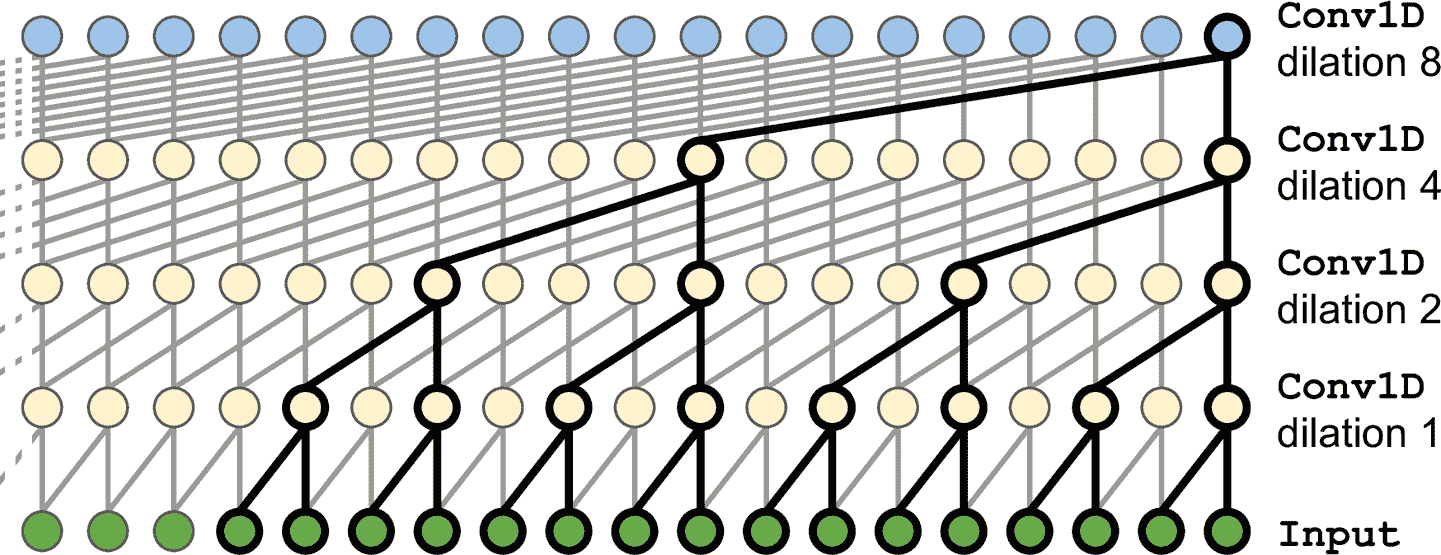model = keras.models.Sequential()
for rate in (1, 2, 4, 8) * 2:
activation="relu", dilation_rate=rate))
history = model.fit(X_train, Y_train, epochs=20,
validation_data=(X_valid, Y_valid))


Sequential模型开头是一个输入层（比只在第一个层上设定input_shape简单的多）；然后是一个 1D 卷积层，使用"causal"填充：这可以保证卷积层在做预测时，不会窥视到未来值（等价于在输入序列的左边用零填充填充合适数量的 0）。然后添加相似的成对的层，膨胀率为 1、2、4、8，接着又是 1、2、4、8。最后，添加输出层：一个有 10 个大小为 1 的过滤器的卷积层，没有激活函数。得益于填充层，每个卷积层输出的序列长度都和输入序列一样，所以训练时的目标可以是完整序列：无需裁剪或降采样。

## 练习

1. 你能说出序列到序列 RNN 的几个应用吗？序列到向量的应用？向量到序列的应用？

2. RNN 层的输入要有多少维？每一维表示什么？输出呢？

3. 如果搭建深度序列到序列 RNN，哪些 RNN 层要设置return_sequences=True？序列到向量 RNN 又如何？

4. 假如有一个每日单变量时间序列，想预测接下来的七天。要使用什么 RNN 架构？

5. 训练 RNN 的困难是什么？如何应对？

6. 画出 LSTM 单元的架构图？

7. 为什么在 RNN 中使用 1D 卷积层？

8. 哪种神经网络架构可以用来分类视频？

9. 为 SketchRNN 数据集（TensorFlow Datasets 中有），训练一个分类模型。

10. 下载 Bach chorales 数据集，并解压。它含有 382 首巴赫作曲的赞美歌。每首的长度是 100 到 640 时间步，每个时间步包含 4 个整数，每个整数对应一个钢琴音符索引（除了 0，表示没有音符）。训练一个可以预测下一个时间步（四个音符）的模型，循环、卷积、或混合架构。然后使用这个模型来生成类似巴赫的音乐，每个时间一个音符：可以给模型一首赞美歌的开头，然后让其预测接下来的时间步，然后将输出加到输入上，再让模型继续预测。或者查看 Google 的 Coconet 模型，它是 Google 来做巴赫曲子的。